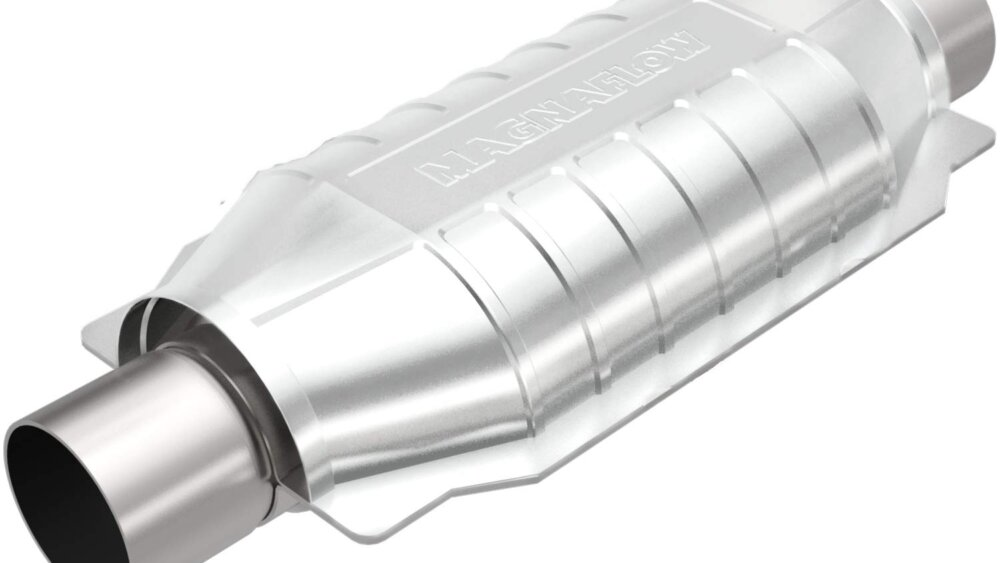# Mengubah Format Datetime Pada Bahasa Python

Subscribe Dengan Account Google Untuk Membaca Artikel Tanpa IklanSeringkali kita menemukan kondisi dimana format tanggal pada pada operating system berbeda dengan format tanggal yang kita harapkan.

Setiap bahasa program memiliki librari converter yang berbeda, untuk bahasa python librari yang banyak digunakana adalah datetime. Datetime merupakan librari bawaan python yang cukup populer.

Pada tutorial kali ini kita akan belajar penggunaan datetime pada 3 studi kasus:

### A. Datetime ke String menggunakan strftime()

##### Perhatikan contoh code di bawah ini:

```from datetime import datetime

now = datetime.now() # current date and time

year = now.strftime("%Y")print("year:", year)

month = now.strftime("%m")print("month:", month)

day = now.strftime("%d")print("day:", day)

time = now.strftime("%H:%M:%S")print("time:", time)

date_time = now.strftime("%m/%d/%Y, %H:%M:%S")print("date and time:",date_time)```

##### Result:

`year: 2022month: 05day: 16time: 09:16:34date and time: 05/16/2022, 09:16:34`

### B. Membuat String Dari Timestamp

##### Perhatikan contoh code dibawah ini:

```from datetime import datetimetimestamp = 1528797322date_time = datetime.fromtimestamp(timestamp)

print("Date time object:", date_time)

d = date_time.strftime("%m/%d/%Y, %H:%M:%S")print("Output 2:", d)

d = date_time.strftime("%d %b, %Y")print("Output 3:", d)

d = date_time.strftime("%d %B, %Y")print("Output 4:", d)

d = date_time.strftime("%I%p")print("Output 5:", d)```

##### Result:

`Date time object: 2018-06-12 16:55:22Output 2: 06/12/2018, 16:55:22Output 3: 12 Jun, 2018Output 4: 12 June, 2018Output 5: 04PM`

### C. Mengubah Timestamp ke Format Date dan Time Local

##### Perhatikan contoh code dibawah ini:

```from datetime import datetime

timestamp = 1528797322date_time = datetime.fromtimestamp(timestamp)

d = date_time.strftime("%c")print("Output 1:", d)

d = date_time.strftime("%x")print("Output 2:", d)

d = date_time.strftime("%X")print("Output 3:", d)```

##### Result::

`Output 1: Tue Jun 12 16:55:22 2018Output 2: 06/12/18Output 3: 16:55:22`

Kode format %c, %x, %X digunakan untuk merepresentasikan format Date dan Time local
##### Berikut ini beberapa code format standar Datetime

 Directive Meaning Example `%a` Abbreviated weekday name. Sun, Mon, ... `%A` Full weekday name. Sunday, Monday, ... `%w` Weekday as a decimal number. 0, 1, ..., 6 `%d` Day of the month as a zero-padded decimal. 01, 02, ..., 31 `%-d` Day of the month as a decimal number. 1, 2, ..., 30 `%b` Abbreviated month name. Jan, Feb, ..., Dec `%B` Full month name. January, February, ... `%m` Month as a zero-padded decimal number. 01, 02, ..., 12 `%-m` Month as a decimal number. 1, 2, ..., 12 `%y` Year without century as a zero-padded decimal number. 00, 01, ..., 99 `%-y` Year without century as a decimal number. 0, 1, ..., 99 `%Y` Year with century as a decimal number. 2013, 2019 etc. `%H` Hour (24-hour clock) as a zero-padded decimal number. 00, 01, ..., 23 `%-H` Hour (24-hour clock) as a decimal number. 0, 1, ..., 23 `%I` Hour (12-hour clock) as a zero-padded decimal number. 01, 02, ..., 12 `%-I` Hour (12-hour clock) as a decimal number. 1, 2, ... 12 `%p` Locale’s AM or PM. AM, PM `%M` Minute as a zero-padded decimal number. 00, 01, ..., 59 `%-M` Minute as a decimal number. 0, 1, ..., 59 `%S` Second as a zero-padded decimal number. 00, 01, ..., 59 `%-S` Second as a decimal number. 0, 1, ..., 59 `%f` Microsecond as a decimal number, zero-padded on the left. 000000 - 999999 `%z` UTC offset in the form +HHMM or -HHMM. `%Z` Time zone name. `%j` Day of the year as a zero-padded decimal number. 001, 002, ..., 366 `%-j` Day of the year as a decimal number. 1, 2, ..., 366 `%U` Week number of the year (Sunday as the first day of the week). All days in a new year preceding the first Sunday are considered to be in week 0. 00, 01, ..., 53 `%W` Week number of the year (Monday as the first day of the week). All days in a new year preceding the first Monday are considered to be in week 0. 00, 01, ..., 53 `%c` Locale’s appropriate date and time representation. Mon Sep 30 07:06:05 2013 `%x` Locale’s appropriate date representation. 09/30/13 `%X` Locale’s appropriate time representation. 07:06:05 `%%` A literal '%' character. %

##### Baca artikel lainya :

sumber referensi : docs.python.org, programiz.com